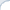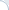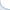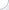I co-authored a a paper on efficient intersection of compressed posting lists. I believe our team obtained good improvements over standard approaches using single instruction multiple data (SIMD) commands available on most modern processors. Here I want to briefly explain what was done and why it was hard to achieve these good improvements.

Parallelization of the algorithms is hard, but parallelization using a single CPU core is even harder. One of the first algorithms that exploited parallel capabilities of a single CPU were bit-parallel algorithms. For instance, if you carry out a bitwise AND between two 32-bit words, you essentially perform 32 boolean ANDs between 32 pairs of variables. Bitwise logical operations are, perhaps, the first SIMD instructions implemented by CPU designers. And thanks to Wikipedia, we know that the first bit-parallel algorithms were proposed more than 40 years ago.

More advanced CPUs may provide a rich set of SIMD instructions (far beyond bit-parallel logical operations). In particular, on x86 CPUs, you can efficiently carry out four additions over four pairs of single-precision floating numbers using a single SIMD instruction. Such instructions operate on small vectors and allow us to significantly improve performance of algorithms that process several streams of data in identical fashion, e.g., matrix multiplication algorithms. Somewhat surprisingly, SIMD operations can accelerate a lightweight compression of integers. By essentially splitting the data set into four parts, one can decompress billions of integers per second.

Unfortunately, CPUs provide only a limited set of control flow operations for vectorized data. In particular, x86-compatible CPUs have an instruction _mm_cmpeq_epi32 that checks element-wise equality of two integer vectors (each of which has four elements). However, this is not sufficient to parallelize an algorithm crucially relying on control flow instructions. Imagine that you compared four pairs of integers using _mm_cmpeq_epi32 and obtained the result in the form of a four-element bitmask (element i is 1 if and only if the the i-th pair have equal numbers). How do you extract matching integers? One clearly needs a gather-scatter instruction, but it is not supported by commodity x86 CPUs.

One devious workaround is to use a shuffle operation. To this end, one needs precomputed tables of shuffle masks. For example, if the comparison results can be interpreted as number 15, you retrieve the shuffle mask 15 from the shuffle-mask table and perform the shuffle operation. Accessing memory is not fast, even if data is in L1 cache. As an example, consider a problem of extracting integer values from sets represented as bitmaps. One well-known solution to this problem involves memoization. However, it is about five times slower than other approaches. If you do not believe, check the performance/code of the function bitscan4 in this sample code.

Now consider a C++ implementation of the classic textbook algorithm to carry out intersections of two sorted lists:

 while (first1!=last1 && first2!=last2)  {    if (*first1<*first2) ++first1;    else if (*first2<*first1) ++first2;    else {      *result = *first1;      ++result; ++first1; ++first2;    }  }

The algorithm has a complexity of $O(n+m)$ and is optimal in many cases ($m$, $n$ are list sizes) . However, it requires a lot of branches, which are often mispredicted. As a result, this algorithm is painfully slow: We estimate its performance to be about 300-400 million integers per second (the number of integers is computed as the sum of list sizes). Clearly, if you have an algorithm that can read a compressed posting list at the speed of 4 billion integers per second, it is not especially useful when the intersection algorithm is so tardy.

Schlegel et al proposed a neat way to vectorize this text-book algorithm. Their approach relies on all-against-all comparison SIMD instruction _mm_cmpestrm. Again, there is no gather-scatter instruction on x86 CPU. So, result extraction relies on the shuffle operation and requires reading of precomputed shuffle masks. Furthermore, even the counting version of this algorithm, which only evaluates the size of the intersection (without saving a result), is not especially efficient. We estimate that performance of this counting version is around 2 billion integers per second. It is much faster than the classic text-book algorithm, but is still an impediment, if the decompression algorithms has the speed of 4 billion integers per second.

More efficient (well-known) intersection algorithms exploit a differential in posting list size: Posting lists have different lengths and the differences are quite substantial. So, one can iterate over a shorter list and check if an element from the shorter list is present in the second list. For instance, the check can be done using a binary search (or a similar in spirit approach). Binary search relies on branching, thus, SIMD instructions should be useless here, right?

I would think so. Yet, Nathan Kurz figured out that it does not have to be the case here. He made a clever observation: When we are carrying out a binary search, eventually the search interval becomes so small that searching this interval sequentially becomes feasible. And such a sequential search can be accelerated using the instruction _mm_cmpeq_epi32 (that compares 4 pairs of integers at a time). This sequential scan can be carried out in a branchless fashion: Comparison results (one result is a bit mask) can be easily aggregated using the SIMD instruction _mm_or_si128. Furthermore, there is no extraction problem here. We iterate over the short list one integer at a time. Whenever, the integer is found in the second list, we memorize this common integer (which we already have in a scalar variable/register). Otherwise, we simply proceed to check the next one.

Nathan and Daniel (I wish I could claim credit here) turned this idea into an efficient intersection algorithm that beats the scalar checking version by a good margin. The performance can be further improved by storing some of the posting lists as bitmaps (the idea proposed by Culpepper and Moffat). As a result of these combined improvements, we achieve a sub-millisecond query-processing time for Gov2. For ClueWeb09 the query-processing time can be less than 2 milliseconds. Yet, I omit further details here and refer the reader to our joint paper.

In conclusion, our approach is based on a simple idea that was hard to come by. It was somewhat counter-intuitive that this simple method would work better than the approach due Schlegel et al, which employs the all-against-all comparison SIMD instruction. In fact, I think that the latter is quite useful when we intersect or merge lists of comparable sizes. However, this is probably not a typical scenario for a textual search engine.